# Measurement & Geometry Basics Chapter Exam

Exam Instructions:

Choose your answers to the questions and click 'Next' to see the next set of questions. You can skip questions if you would like and come back to them later with the yellow "Go To First Skipped Question" button. When you have completed the practice exam, a green submit button will appear. Click it to see your results. Good luck!

### Page 1

#### Question 2 2. What is the line, as shown in the picture, called?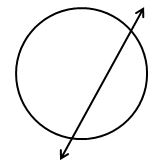#### Question 3 3. Given the triangle PQE, calculate the measure of Angle Q.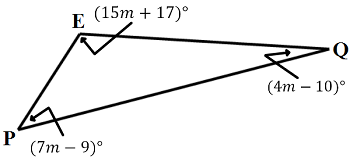### Page 2

#### Question 6 6. What is shown below?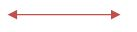### Page 3

#### Question 14 14. In the figure below, Angle 7 = 15x and Angle 10 = 9x + 30. Determine the measure of Angle 10.### Page 4

#### Question 17 17. You are building a raised plant bed and need to know the area of the bed in order to cover it with planters paper to keep the weeds out. What is the area of your plant bed?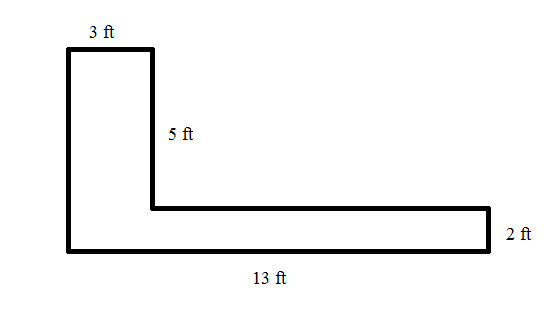#### Question 18 18. These polygons belong to which categories? A: Regular B: Convex C: Concave D: Irregular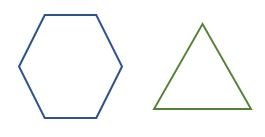#### Question 19 19. Find the surface area. Assume that the line labeled 5 represents the radius of the cone's base.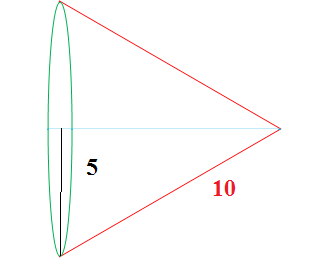#### Question 20 20. Given the triangle ABC, solve for the value of y.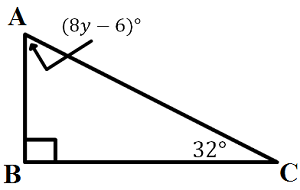### Page 5

#### Question 21 21. Find the perimeter.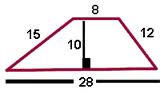#### Question 22 22. What shape is the candle in this candle holder?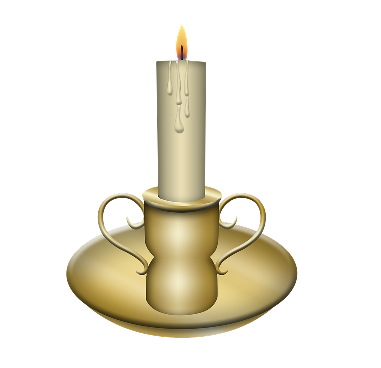### Page 6

#### Question 28 28. In triangle GBN, Angle G is seven more than three times a number, Angle B is five more than four times the number, and Angle N is twelve less than five times the number. Solve for the measure of Angle G.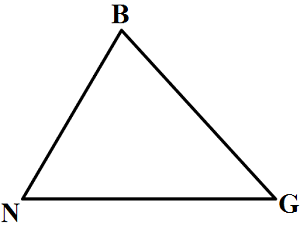#### Question 29 29. What is the perimeter?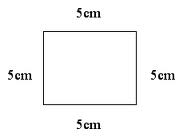#### Measurement & Geometry Basics Chapter Exam Instructions

Choose your answers to the questions and click 'Next' to see the next set of questions. You can skip questions if you would like and come back to them later with the yellow "Go To First Skipped Question" button. When you have completed the practice exam, a green submit button will appear. Click it to see your results. Good luck!

Support